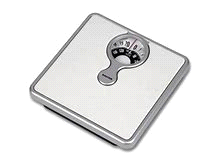# Lesson Notes By Weeks and Term - Primary 3

Measurement of mass

WEEK 2

Subject: Basic Science

Class: Primary 3

Term: 1st Term

Topic: Measurement of mass

Subtitle: Mass

Learning Objectives: At the end of this this lesson, pupils should be able to:

1. Define mass
2. State two instruments for measuring mass
3. State the unit of mass

Resources and materials:

Scheme of work

Online information

Instructional material: Kitchen Scale, weighing balance and beam balance

Building Background/connection to prior knowledge: pupils are familiar with the topic in their previous classes.

CONTENT

Mass is the amount weight a body or and contains. We can use the following to know the mass of an object; beam balance, kitchen scale, spring balance and bathroom scale.Beam balance      Kitchen scale

We know the main standard unit of mass or weight is kilogram which we write in short as ‘kg’. 1000th part of this kilogram is gram which is written in short as ‘g’. Thus 1000 gram = 1kilogram and 1 kilogram = 1000 gram

Below is a picture of objects of different weight, 500g and 1kg.Strategies& Activities:

Step1: Teacher revises the previous topic.

Step2: Teacher introduces the new topic.

Step3: Teacher explains the new topic.

Step4: Teacher welcomes pupils’ questions.

Step5: Teacher evaluates the pupils.

Assessment & Evaluation: 1. What is mass?

1. mention two instrument you can use to measure mass.

WRAP UP(CONCLUSION) Teacher goes over the topic once again for better understanding.

Assignment:1. Convert 9kg to grams

2. write three instruments we can use to measure mass